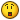## Recommended Posts

So I've had this durdge of probable unecessary extra lines in one of my scripts for a while, mainly due to my inability to wrap my brain around a way to loop a section of code so it cycles variables the way I need, so it doesn't break the other 3000 lines. Let me preface the showing of this code by saying this was one of the first scripts I wrote in AutoIt, so please be kind... I don't make these kinds of mistakes... often.If I did a total rewrite of the script, it'd look quite different now.

I do the following, 30 times, with certain variables changing names in each section... When you look at the code, note that the only variables that change have "gaat" in their names. They change as a location code for each site, such as "gane" or "gase", again there's 30 different versions of those variables. I know there must be an easy way to do a loop so I only have to do it once, anyways, here's the code:

```; Define variables
Dim \$gaat
Dim \$filename
Dim \$agaatLine

; Read UND Data From GAAT
\$filename = FileOpen("C:\undsearch\gaat-und.csv", 0)

; Check if file opened for reading OK
If \$filename = -1 Then
MsgBox(0, "Error", "Unable to open GAAT file.")
Exit
EndIf

; Read in lines of text until the EOF is reached
DIM \$acounti
\$counti = 0
DIM \$resultcount
\$resultcount = -1
While 1
\$counti = \$counti + 1
If @error = -1 Then ExitLoop
\$stringfound = stringInstr(\$gaatline, \$racfid)
If \$stringfound > 0 THEN
\$resultcount = \$resultcount+1
_ArrayInsert( \$acounti, \$resultcount, \$counti)
EndIf
\$stringfound = 0
Wend
IF IsArray(\$acounti) THEN
IF \$acounti>0 THEN
\$agaatLine1 = StringSplit(\$gaatline,"|")
EndIf
IF \$acounti>0 THEN
\$agaatLine2 = StringSplit(\$gaatline,"|")
EndIf
IF \$acounti>0 THEN
\$agaatLine3 = StringSplit(\$gaatline,"|")
EndIf
IF \$acounti>0 THEN
\$agaatLine4 = StringSplit(\$gaatline,"|")
EndIf
IF \$acounti>0 THEN
\$agaatLine5 = StringSplit(\$gaatline,"|")
EndIf
IF \$acounti>0 THEN
\$agaatLine6 = StringSplit(\$gaatline,"|")
EndIf
IF \$acounti>0 THEN
\$agaatLine7 = StringSplit(\$gaatline,"|")
EndIf
IF \$acounti>0 THEN
\$agaatLine8 = StringSplit(\$gaatline,"|")
EndIf
IF \$acounti>0 THEN
\$agaatLine9 = StringSplit(\$gaatline,"|")
EndIf
IF \$acounti>0 THEN
\$agaatLine10 = StringSplit(\$gaatline,"|")
EndIf
EndIf
FileClose(\$filename)```

If anyone has a suggestion for a relatively painless method of looping the affected variables, I'm willing to listen. If I could insert variable names inside of an array for use later, this would be much easier... or can I? I've never seen an example of this, so I'm not sure how you'd go about it.

-Crim

##### Share on other sites

IF \$acounti>0 THEN

\$agaatLine1 = StringSplit(\$gaatline,"|")

EndIf

IF \$acounti>0 THEN

\$agaatLine2 = StringSplit(\$gaatline,"|")

EndIf

IF \$acounti>0 THEN

\$agaatLine3 = StringSplit(\$gaatline,"|")

EndIf

IF \$acounti>0 THEN

\$agaatLine4 = StringSplit(\$gaatline,"|")

EndIf

IF \$acounti>0 THEN

\$agaatLine5 = StringSplit(\$gaatline,"|")

EndIf

IF \$acounti>0 THEN

\$agaatLine6 = StringSplit(\$gaatline,"|")

EndIf

IF \$acounti>0 THEN

\$agaatLine7 = StringSplit(\$gaatline,"|")

EndIf

IF \$acounti>0 THEN

\$agaatLine8 = StringSplit(\$gaatline,"|")

EndIf

IF \$acounti>0 THEN

\$agaatLine9 = StringSplit(\$gaatline,"|")

EndIf

IF \$acounti>0 THEN

\$agaatLine10 = StringSplit(\$gaatline,"|")

EndIf

if i'm not mistaken you could simplify this as follows.. not sure if its exactly what you're looking for but its generally more tidy

try this:

```dim \$i

dim \$agaatline
\$agaatline = _ArrayCreate(StringSplit(\$gaatline,"|"))
select case \$acounti
for \$i = 1 to 10
\$agaatLine[\$i] = StringSplit(\$gaatline,"|")
Next```

im not sure about the \$agaatLine bit, but the 1st line at least will work i think

Peace

Edited by Sarc
##### Share on other sites

if i'm not mistaken you could simplify this as follows.. not sure if its exactly what you're looking for but its generally more tidy

try this:

```dim \$i

dim \$agaatline
\$agaatline = _ArrayCreate(StringSplit(\$gaatline,"|"))
select case \$acounti
for \$i = 1 to 10
\$agaatLine[\$i] = StringSplit(\$gaatline,"|")
Next```

im not sure about the \$agaatLine bit, but the 1st line at least will work i think

Peace

StringSplit creates an array, so passing it to _ArrayCreate() i don't think would work the way that you intend.

this is untested, but it may work for you, although i think that creating an array instead of having 10 different gaat variables would be the way to go...

```If IsArray(\$acounti) Then
For \$iterator = 0 To 9
If \$acounti[\$iterator] > 0 Then
Assign("\$agaatLine" & \$iterator, StringSplit(\$gaatline, "|")
EndIf
Next
FileClose(\$filename)
EndIf```
##### Share on other sites

Thanks you all, that worked pretty well... I had a few small typos to fix, but the following worked perfectly... and I learned a new function! (Assign)

Here's the final code that worked:

```If IsArray(\$acounti) Then
For \$iterator = 0 To 9 Step 1
If \$acounti[\$iterator] > 0 Then
Assign("agaatLine" & \$iterator, StringSplit(\$gaatline, "|"))
EndIf
Next
FileClose(\$filename)
EndIf```
##### Share on other sites

Thanks you all, that worked pretty well... I had a few small typos to fix, but the following worked perfectly... and I learned a new function! (Assign)

Here's the final code that worked:

```If IsArray(\$acounti) Then
For \$iterator = 0 To 9 Step 1
If \$acounti[\$iterator] > 0 Then
Assign("agaatLine" & \$iterator, StringSplit(\$gaatline, "|"))
EndIf
Next
FileClose(\$filename)
EndIf```
glad to help. so you know, the default step is 1, so adding that is kind of redundant...

## Create an account

Register a new account

• ### Recently Browsing   0 members

×

• Wiki

• Back

• #### Beta

• Git
• FAQ
• Our Picks
×
• Create New...